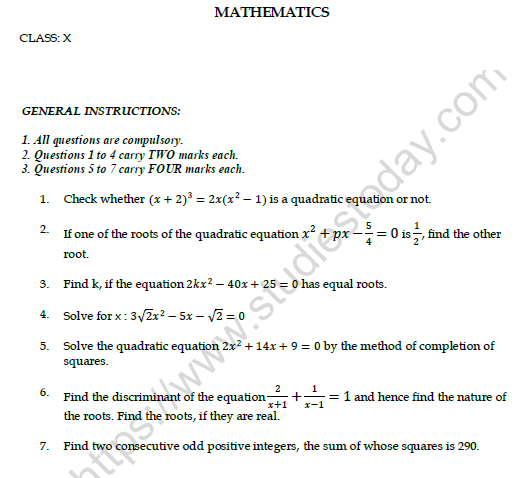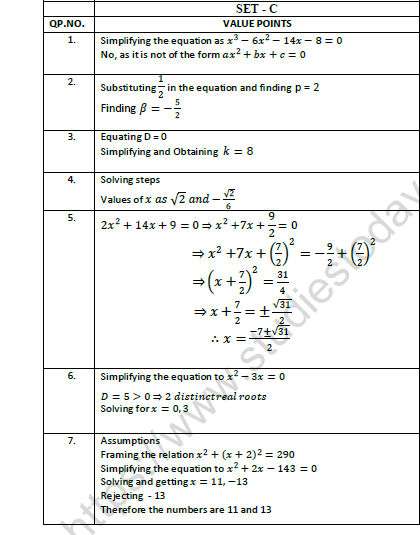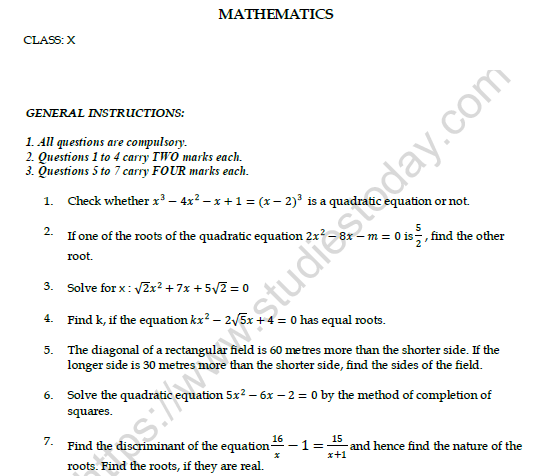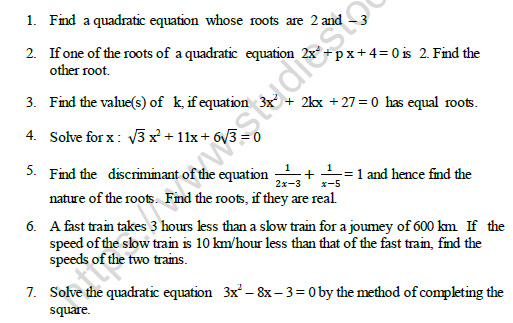# Class 10 Mathematics Printable Worksheets

• ### CBSE Class 10 Mathematics Worksheet Set F

SECTION :A   1. For what values of k, do the following pair of linear equations have infinitely many solutions?       kx + 3y = k -3 and 12x + ky = k .   2. The difference between two numbers is 26. If one number is thrice the other, find the numbers. 2   3. Name the type of lines, the following...

• ### CBSE Class 10 Mathematics Worksheet Set E

SECTION: A   1. The difference between two numbers is 26. If one number is thrice the other, find the numbers.    2. Solve for x and y algebraically: 2x – 3y = -4, 5x + y = 7    3. Name the type of lines, the following pair of linear equations represents. Justify your answer:   i. 2x + 3y = 4 ; 2x...

• ### CBSE Class 10 Mathematics Worksheet Set D

SECTION: A   1. Name the type of lines, the following pair of linear equations represents. Justify your answer:   i. 2x + 3y = 4 ; 2x - 3y = 4                ii. x - 2y = 1 ; 3x - 6y = 5   2. For what values of k, do the following pair of linear equations have infinitely many solutions?   kx + 3y...

# CBSE Class 10 Mathematics Worksheet Set F

Click for more Mathematics Study Material
 CBSE Class 10 Mathematics Question Bank CBSE Class 10 Mathematics Worksheet Set A Solved CBSE Class 10 Mathematics Worksheet Set B Solved CBSE Class 10 Mathematics Worksheet Set C Solved CBSE Class 10 Mathematics Worksheet Set D CBSE Class 10 Mathematics Worksheet Set E CBSE Class 10 Mathematics Worksheet Set F

# CBSE Class 10 Mathematics Worksheet Set E

Click for more Mathematics Study Material
 CBSE Class 10 Mathematics Question Bank CBSE Class 10 Mathematics Worksheet Set A Solved CBSE Class 10 Mathematics Worksheet Set B Solved CBSE Class 10 Mathematics Worksheet Set C Solved CBSE Class 10 Mathematics Worksheet Set D CBSE Class 10 Mathematics Worksheet Set E CBSE Class 10 Mathematics Worksheet Set F

# CBSE Class 10 Mathematics Worksheet Set D

Click for more Mathematics Study Material
 CBSE Class 10 Mathematics Question Bank CBSE Class 10 Mathematics Worksheet Set A Solved CBSE Class 10 Mathematics Worksheet Set B Solved CBSE Class 10 Mathematics Worksheet Set C Solved CBSE Class 10 Mathematics Worksheet Set D CBSE Class 10 Mathematics Worksheet Set E CBSE Class 10 Mathematics Worksheet Set F

# CBSE Class 10 Mathematics Worksheet Set C SolvedClick for more Mathematics Study Material
 CBSE Class 10 Mathematics Question Bank CBSE Class 10 Mathematics Worksheet Set A Solved CBSE Class 10 Mathematics Worksheet Set B Solved CBSE Class 10 Mathematics Worksheet Set C Solved CBSE Class 10 Mathematics Worksheet Set D CBSE Class 10 Mathematics Worksheet Set E CBSE Class 10 Mathematics Worksheet Set F

# CBSE Class 10 Mathematics Worksheet Set B SolvedClick for more Mathematics Study Material
 CBSE Class 10 Mathematics Question Bank CBSE Class 10 Mathematics Worksheet Set A Solved CBSE Class 10 Mathematics Worksheet Set B Solved CBSE Class 10 Mathematics Worksheet Set C Solved CBSE Class 10 Mathematics Worksheet Set D CBSE Class 10 Mathematics Worksheet Set E CBSE Class 10 Mathematics Worksheet Set F

# CBSE Class 10 Mathematics Worksheet Set A SolvedClick for more Mathematics Study Material
 CBSE Class 10 Mathematics Question Bank CBSE Class 10 Mathematics Worksheet Set A Solved CBSE Class 10 Mathematics Worksheet Set B Solved CBSE Class 10 Mathematics Worksheet Set C Solved CBSE Class 10 Mathematics Worksheet Set D CBSE Class 10 Mathematics Worksheet Set E CBSE Class 10 Mathematics Worksheet Set F

# CBSE Class 10 Mathematics Question Bank

Click for more Mathematics Study Material
 CBSE Class 10 Mathematics Question Bank CBSE Class 10 Mathematics Worksheet Set A Solved CBSE Class 10 Mathematics Worksheet Set B Solved CBSE Class 10 Mathematics Worksheet Set C Solved CBSE Class 10 Mathematics Worksheet Set D CBSE Class 10 Mathematics Worksheet Set E CBSE Class 10 Mathematics Worksheet Set F

## Latest NCERT & CBSE News

Read the latest news and announcements from NCERT and CBSE below. Important updates relating to your studies which will help you to keep yourself updated with latest happenings in school level education. Keep yourself updated with all latest news and also read articles from teachers which will help you to improve your studies, increase motivation level and promote faster learning

### CBSE Term 2 Board Examinations

CBSE vide Circular No.Acad-51/2021 dated 5th July, 2021, notified that in the session 2021-2022, Board Examinations would be conducted in two terms, i.e.. Term I and Term II. This decision was taken due to the uncertainty arising out of COVID 19 Pandemic. Term I...

### Online courses for classes XI and XII offered by NCERT

Ministry of Education (MoE), Government of India has launched a platform for offering Massive Open Online Courses (MOOCs) that is popularly known as SWAYAM (Study Webs of Active learning for Young Aspiring Minds) on 9 th July, 2017. NCERT now offers online courses for...

### Celebration of Matribhasha Diwas Mother Language day

UNESCO has declared 21st February of every year to be celebrated as International Mother Language day to promote dissemination of Mother Language of all, create awareness of linguistic and cultural traditions and diversity across the world and to inspire solidarity...

### CBSE Science Challenge 2021 22

Science is inexplicably linked with our lives and helps us to understand the world around us better. Scientific and technological developments contribute to progress and help improve our standards of living. By engaging with this subject, students learn to think, solve...

### Heritage India Quiz 2021 2022

CBSE Heritage India Quiz is conducted every year to raise the awareness about the preserving human heritage, diversity and vulnerability of the India's built monuments and heritage sites. It is an attempt of the Board to motivate the future generations of this country...

### Surya Namaskar Project on 75th Anniversary of Independence Day

Ministry of Education, Govt of India vide letter No. F.No. 12-5/2020-IS-4 dated 16.12.2021 has intimated that under the banner Azadi ka Amrit Mahotsav the National Yogasanasports Federation has decided to run a project of 750 million Surya Namaskar from 01 January 2022...